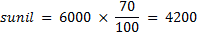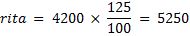## Percentage For SBI PO : Set – 08

1) 5000 students appeared in an examination in which 1/5 of the total students are girls and remaining are boys. 18% boys and 28% girls are failed. What is the percentage of passed students?

a) 85

b) 86

c) 88

d) 80

e) None of these

d)

Total number of student = 5000

Total girls = 1/5 parts

Total boys= 4000   total girls= 1000

Boys failed= 18%    Girls failed= 28%

Boys Passed = 4000-720= 3280

Girls passed = 1000-280= 720

Total Passed =(4000/5000) *100=80

2) 7% of the total quantity of wheat is lost in grinding when a country has to import 12 million tonnes, but when only 5 1/5% is lost, it can import 3 million tonnes. Find the quantity of wheat grown in the country.

a) 100 million tonnes

b) 500 million tonnes

c) 600 million tonnes

d) 400 million tonnes

e) 300 million tonnes

b)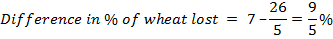Difference in import = 12 – 3 = 9 million As 9/5% of total qty of wheat = 9 million

-> 9x/500=9

=> x = 500 million.

3) The total tractor population in a state is 2, 95, 00 out of which 1, 50, 00 are made by Mahindra & Mahindra. Out of every 100 Mahindra tractors, 98 are red in colour, but only 53% of the total tractor population is red. Find the percentage of non- Mahindra tractors that are red.

a) 5.025%

b) 5.130%

c) 6.125%

d) 6.440%

e) 7.145%

d)

Total Tractor = 29500

Mahindra Tractor = 15000

Non Mahindra Tractor = 29500-15000 =    14500

53% of 29500 = 15635

Non red Mahindra = 15635 – ( 98*15000/100)

Non red Mahindra = 935

% of non Mahindra =  (935/14500)*100= 6.4

4) In an election contested by two parties, Party D secured 12% of the total votes more than Party R. If party R got 132,000 votes, by how many votes did it lose the election?

a) 240,000

b) 300,000

c) 168,000

d) 36,000

e) 24,000

d)

Let the percentage of the total votes  secured by party D be x%

Then the percentage of total votes secured by Party R = (x-12) %

As there are only two parties contesting in the election, the sum total of the votes secured by the two parties should total up to 100%

i.e.,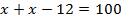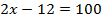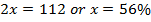If party D got 56% of the votes, then party got (56-12) =44% of the total votes.

i.e.,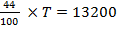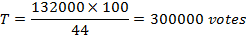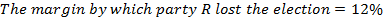300000=36000

5) The population of city X increased from 650, 000 in 2000 to 700, 000 in 2010, and it is projected that the population will increase by the same number from 2010 to 2020. Approximately what is the projected percent increase in population from 2010 to 2020 ?

a) 7. 1%

b) 7. 7%

c) 8. 3%

d) 14. 3%

e) 15. 3%

a)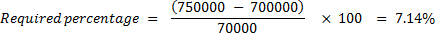6) If the price of commodity be raised by 18 2/3 %, find by how much percent must a householder reduce his consumption of that commodity, so as not to increase his expenditure?

a) 14%

b) 15. 73%

c) 12%

d) 13. 25%

e) 16. 43%

b)

Required reduction in consumption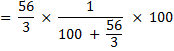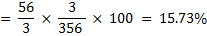7) Nehru borrowed some money at the rate of 6% per annum for the first 3 yr, at the rate of 9% per annum for the next 5 yr and at the rate of 13% per annum for the period beyond 8 yr. If he pays a total interest of Rs. 8160 at the end of 11 yr, how much money did he borrow?

a) 12000

b) 10000

c) 8000

e) None of the above.

c)

Let the sum borrowed be P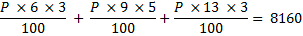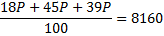P=8000.

8) The largest and second largest angles of a triangle are in the ratio of  3 : 2 respectively. The smallest angle is 20% of the sum of the largest and second largest angles. What is the sum of the smallest and second largest angles?

a) 80

b) 60

c) 100

d) 90

e) None of these

d)

largest : second largest = 3: 2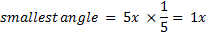sum of three angle = 180

6x= 180

x = 30

smallest + second largest = 3x = 90

9) In a college 12 % of total students are interested in sports.3/4th of total students are interested in dance. 10% of total students are interested in singing and remaining 15 students are not interested in any activity. How many students are there in the college?

a) 450

b) 650

c) 500

d) 600

e) cannot be determined

c)

12 % + 75 % +10% = 97 % students particpate

remaining 3 % not participate

3 % = 15

then 100 % = 500

10) Rita invested 25% more than sunil. Sunil invested 30% less than Abhinav who invested rs 6000 . What is the respective ratio between the amount that Rita invested and the total amount invested by all of them together?

a) 35 : 104

b) 13 : 29

c) 101 : 36

d) 35 : 103

e) None of these